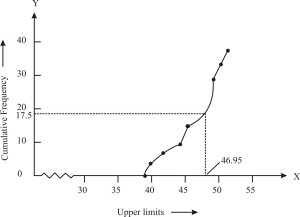# During the medical check-up of 35 students of a class, their weights were recorded as follows: Draw a less than type ogive for the given data. Hence obtain the median weight from the graph and verify the result by using the formula.

 Weight in kg Number of students Less than 38 0 Less than 40 3 Less than 42 5 Less than 44 9 Less than 46 14 Less than 48 28 Less than 50 32 Less than 52 35

Solution: From the given parameters, to represent the table in the form of a graph, we will choose the upper limits of the class intervals are in x-axis and frequencies on the y-axis by choosing the convenience scale.

On plotting the points corresponding to the ordered pairs given by

(38, 0), (40, 3), (42, 5), (44, 9),(46, 14), (48, 28), (50, 32) and (52, 35) on a graph paper an join them to get a smooth curve. The curve obtained is known as less than type ogive.Now will locate 17.5 on the y-axis and draw a line parallel to the x-axis cutting the curve at a point. From the point, draw a perpendicular line to the x-axis. The intersection point perpendicular to the x-axis is the median of the given data. Now, to find the mode by making a table.

 Class interval Number of students(Frequency) Cumulative Frequency Less than 38 0 0 Less than 40 3-0=3 3 Less than 42 5-3=2 8 Less than 44 9-5=4 9 Less than 46 14-9=5 14 Less than 48 28-14=14 28 Less than 50 32-28=4 32 Less than 52 35-22=3 35

Determination of mode

$$l+(\frac{f_{1}-f_{0}}{2f_{1}-f_{0}-f_{2}})\times h$$

The class 46 – 48 has the maximum frequency,

Hence, = 46, h = 2, f1= 14, f0= 5 and f2 = 4

Mode = 46 + 0.95 = 46.95

Thus, mode is verified.